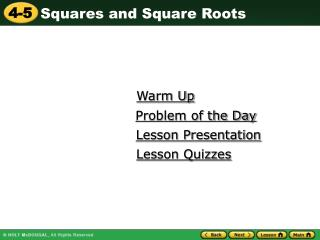DownloadDownload PresentationWarm Up

# Warm Up

Télécharger la présentation## Warm Up

- - - - - - - - - - - - - - - - - - - - - - - - - - - E N D - - - - - - - - - - - - - - - - - - - - - - - - - - -
##### Presentation Transcript

1. Warm Up Problem of the Day Lesson Presentation Lesson Quizzes

2. Warm Up Simplify. 1. 522. 82 64 25 225 144 3. 1224. 152 400 5. 202

3. Problem of the Day A Shakespearean sonnet is a poem made up of 3 quatrains (4 lines each), and a couplet (2 lines). Each line is in iambic pentameter (which means it has 5 iambic feet). So, how many iambic feet long is a Shakespearean sonnet? 70

4. Sunshine State Standards MA.8.A.6.2 Make reaonable approximations of square roots…,and use them to estimate solutions to problems…

5. Vocabulary square root principal square root perfect square

6. 62 = 36 36 = 6 Think about the relationship between the area of a square and the length of one of its sides. area = 36 square units side length = 6 units because 62=36 A number that when multiplied by itself to form a product is the square rootof that product. Taking the square root of a number is the inverse of squaring the number.

7. Caution! –49 is not the same as – 49. A negative number has no real square root. Every positive number has two square roots, one positive and one negative. The radical symbol indicates the nonnegative or principal square root. The symbol – is used to indicate the negative square root. The numbers 16, 36, and 49 are examples of perfect squares. A perfect square is a number that has integers as its square roots. Other perfect squares include 1, 4, 9, 25, 64, and 81.

8. 49 = 7 49 = –7 – 100 = 10 100 = –10 – 225 = 15 225 = –15 – Additional Example: 1 Finding the Positive and Negative Square Roots of a Number Find the two square roots of each number. A. 49 7 is a square root, since 7 • 7 = 49. –7 is also a square root, since –7 • –7 = 49. B. 100 10 is a square root, since 10 • 10 = 100. –10 is also a square root, since –10 • –10 = 100. C. 225 15 is a square root, since 15 • 15 = 225. –15 is also a square root, since –15 • –15 = 225.

9. Check It Out: Example 1 Find the two square roots of each number. A. 81 9 • 9 = 81 81 = (–9)(–9) = 81 = ±9 B. 144 144 = 12 • 12 = 144 (–12)(–12) = 144 = ±12 C. 324 324 = 18 • 18 = 324 (–18)(–18) = 324 = ±18

10. So 169 = 13. Remember! The area of a square is s2, where s is the length of a side. Additional Example 2: Application A square window has an area of 169 square inches. How wide is the window? Write and solve an equation to find the area of the window. 132 = 169 Use the positive square root; a negative length has no meaning. The window is 13 inches wide.

11. 225 = 15; Check It Out: Example 2 A square window has an area of 225 square inches. How wide is the window? 152 = 225 so The window is 15 inches wide.

12. 3 36 + 7 3 36 + 7 = 3(6) + 7 Additional Example 3A: Evaluating Expressions Involving Square Roots Simplify the expression. Evaluate the square root. = 18 + 7 Multiply. = 25 Add.

13. 25 16 3 4 3 4 25 16 25 16 3 4 = 1.5625. 1.5625 + = + Additional Example 3B: Evaluating Expressions Involving Square Roots Simplify the expression. + 3 4 Evaluate the square roots. = 1.25 + = 2 Add.

14. 2 121 + 9 2 121 + 9 = 2(11) + 9 Check It Out: Example 3A Simplify each expression. = 22 + 9 = 31

15. 16 36 2 3 1636 2 3 + Check It Out: Example 3B Simplify each expression. + 16 2 3 = + 36 2 3 = + 4 6 1 3 4 3 = , or 1

16. –5 336 + 25 –5 336 + 25 = –5 361 Check It Out: Example 3C Simplify each expression. = –5(19) = –95

17. 2 48 12 • 12 • = 2 48 • 12 2 48 2 576 Check It Out: Example 3D Simplify each expression. = = 2(24) = 48

18. Lesson Quizzes Standard Lesson Quiz Lesson Quiz for Student Response Systems

19. Lesson Quiz Find the two square roots of each number. 1. 81 2. 2500 Evaluate each expression. 3. 3 16 + 1 4. 7 9 – 2 49 9 50 7 13 5. Ms. Estefan wants to put a fence around 3 sides of a square garden that has an area of 225 ft2. How much fencing does she need? 45 ft

20. Lesson Quiz for Student Response Systems 1. Find two square roots of each number. 64 A. 4 B. 8 C.9 D.16

21. Lesson Quiz for Student Response Systems 2. Find two square roots of each number. 6400 A. 4 B. 8 C.80 D.800

22. Lesson Quiz for Student Response Systems 3. Evaluate the expression. A. 44 B. 17 C.19 D.72

23. Lesson Quiz for Student Response Systems 4. Evaluate the expression. A. 4 B. 8 C.16 D.40